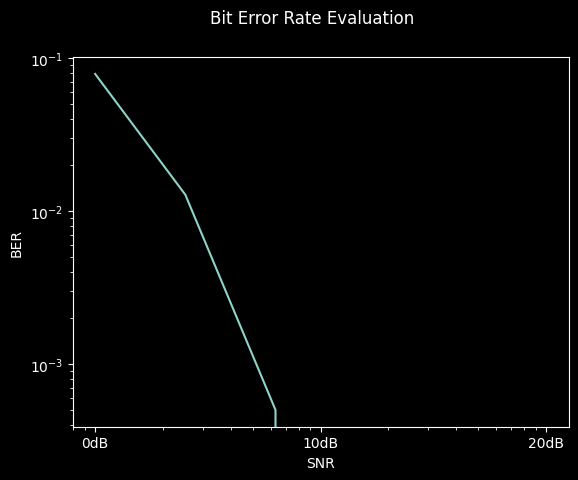Note

This static document was automatically created from the output of a jupyter notebook.

Execute and modify the notebook online here.

# Implementing FEC codings#

This Jupyter notebook will outline the step-by-step process of implementing a new coding scheme for the forward error correction in communication modems. The selected algorithm is a repetition encoder, since it is arguably the most basic error correction coding.

As an initial step, we will import all required modules from HermesPy:

:

import numpy as np
from hermespy.fec import Encoder


Error correcting coding steps are represented by the abstract Encoder interface. Each coding algorithm is represented by a class inheriting from the interface, which requires them to implement the abstract functions and properties

Let’s assume our repetition coding takes blocks of $$K = 4$$ data bits and repeats them $$3$$ times. The resulting code block size would be $$N = 3K = 12$$, which results in a rate of \begin{equation} \mathbf{R} = \frac{K}{N} = \frac{1}{3} \end{equation} for the full encoding. During decoding, the repetition coding decides by majority voting which bit has been transmitted. So, our coding implementation is

:

class RepetitionCoding(Encoder):

@property
def bit_block_size(self) -> int:
return 4

@property
def code_block_size(self) -> int:
return 12

def encode(self, data: np.ndarray) -> np.ndarray:
return np.tile(data, 3)

def decode(self, code: np.ndarray) -> np.ndarray:
return (np.mean(np.reshape(code, (3, self.bit_block_size)), axis=0, keepdims=False) > .5).astype(int)


Now we can inspect our coding implementation:

:

coding = RepetitionCoding()
print(f"Our coding rate is {coding.rate} with an input block size of {coding.bit_block_size} and an output block size of {coding.code_block_size}")

data = np.random.randint(0, 2, coding.bit_block_size)
print(f"Let's assume we transmit the following data block: {data}")

code = coding.encode(data)
print(f"After encoding the respective code block is: {code}")

error_code = code.copy()
error_code = not error_code
print(f"After channel propagation the first bit has flipped: {error_code}")

corrected_data = coding.decode(error_code)
print(f"But the coding can correct a single bit flip to: {corrected_data}")

Our coding rate is 0.3333333333333333 with an input block size of 4 and an output block size of 12
Let's assume we transmit the following data block: [1 0 1 0]
After encoding the respective code block is: [1 0 1 0 1 0 1 0 1 0 1 0]
After channel propagation the first bit has flipped: [0 0 1 0 1 0 1 0 1 0 1 0]
But the coding can correct a single bit flip to: [1 0 1 0]


We may now investigate our newly created forward error correction coding within a full Hermes simulation capaign.

:

from hermespy.core import ConsoleMode, dB
from hermespy.simulation import Simulation
from hermespy.modem import BitErrorEvaluator, DuplexModem, RootRaisedCosineWaveform

# Create a new simulation featuring a single device transmitting at 10GHz
simulation = Simulation(console_mode=ConsoleMode.SILENT)
device = simulation.scenario.new_device(carrier_frequency=10e9)

# Configure a communication operation on the device, using our coding
modem = DuplexModem()
modem.waveform_generator = RootRaisedCosineWaveform(modulation_order=4, oversampling_factor=2, num_preamble_symbols=0, num_data_symbols=10, symbol_rate=1e6)
modem.device = device

# Run a very low-demanding simulation for demonstration purposes
simulation.new_dimension('snr', dB(0, 4, 8, 16, 18, 20))
simulation.num_samples = 1000

result = simulation.run()


This allows us to visualize the achieved bit error rates for given linear signal to noise ratios:

:

_ = result.plot()Exception in thread ray_listen_error_messages:
Traceback (most recent call last):
File "C:\Users\Stealth\AppData\Local\Programs\Python\Python310\lib\threading.py", line 1016, in _bootstrap_inner
2023-07-12 12:17:21,973 ERROR worker.py:914 -- print_logs: <_MultiThreadedRendezvous of RPC that terminated with:
status = StatusCode.UNKNOWN
details = "Stream removed"
debug_error_string = "UNKNOWN:Error received from peer ipv4:127.0.0.1:61812 {grpc_message:"Stream removed", grpc_status:2, created_time:"2023-07-12T10:17:21.971775063+00:00"}"
>
status = StatusCode.UNKNOWN
details = "Stream removed"
debug_error_string = "UNKNOWN:Error received from peer ipv4:127.0.0.1:61812 {grpc_message:"Stream removed", grpc_status:2, created_time:"2023-07-12T10:17:21.971759664+00:00"}"
>
self.run()
File "C:\Users\Stealth\AppData\Local\Programs\Python\Python310\lib\threading.py", line 953, in run
self._target(*self._args, **self._kwargs)
File "d:\envs\hermes-310\lib\site-packages\ray\_private\worker.py", line 2004, in listen_error_messages
_, error_data = worker.gcs_error_subscriber.poll()
File "d:\envs\hermes-310\lib\site-packages\ray\_private\gcs_pubsub.py", line 300, in poll
self._poll_locked(timeout=timeout)
File "d:\envs\hermes-310\lib\site-packages\ray\_private\gcs_pubsub.py", line 217, in _poll_locked
fut.result(timeout=1)
File "d:\envs\hermes-310\lib\site-packages\grpc\_channel.py", line 744, in result
raise self